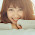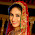Friday, July 15, 2011

List of useful Regular expressions (regex)

Positive integers

^[1-9]+[0-9]*\$

Positive decimal values

(^\d*\.?\d*[0-9]+\d*\$)|(^[0-9]+\d*\.\d*\$)

Percentage (2 decimal places)

^-?[0-9]{0,2}(\.[0-9]{1,2})?\$|^-?(100)(\.{1,2})?\$

List of semi-colon seperated email addresses

^([\w\.*\-*]+@([\w]\.*\-*)+[a-zA-Z]{2,9}(\s*;\s*[\w\.*\-*]+@([\w]\.*\-*)+[a-zA-Z]{2,9})*)\$

German Date (dots instead of slashes)

^(((0[1-9]|\d|3).(0|1).(\d{2}))|((0[1-9]|\d|30).(0|1).(\d{2}))|((0[1-9]|1\d|2[0-8]).02.(\d{2}))|(29.02.((0||)|(00))))\$

1.2.